# Using Logistic Regression for Image Classification

Machine Learning Project

# SYSTEM REQUIREMENTS

• Basic python programming skills
• Basic knowledge of logistic regression
• Numpy python library
• Pandas python library
• Matplotlib python library

Before anything else add this lines of code at the top of your file

In case you need it you can use the command “pip install package_name” to set up your work environment.

# PROBLEM STATEMENT

The idea of this project is to develop and train a model that is able to take the pixel values of a digit and identify if it is an image of the digit one or not.

# SET UP OF DATA

Here you can download the dataset

Each row of the dataset represents the flattened pixel values of a digit, it goes from zero to nine but we will not use all of them because the goal of the binary classifiction model is return 1 if the digit is one and 0 otherwise.

To retrieve the digits data (X) and labels (Y) we must add in our code the following lines:

Because this model will identify the digit 1 only, in the labels we need to convert the rest of digits that are not one to zero.

From the 5000 rows of data, 4000 will be used to train the model and the remaining 1000 will be used to test it, because is important for the model to be tested by unseen data. To do this we must add the following lines to our code:

These training and test datasets are in DataFrame form so they need to be in an array format for the convenience of calculation. Also it is worth noting that we are using .T to take the transpose of each dataset.

Once the training and test datasets are ready to be used it is time to focus on the model itself. To give it form we must define some formulas.

# FORMULAS

We start from the basic linear regression formula: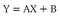Formula 1

Where Y is the output, X is the independent variable, A is the slope and B is the intercept. But in logistic regression variables are expressed diferrently: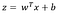Formula 2

Where:

• z is the output variable
• x is the input variable
• w and b will be initialized as zeros and they will be modified while training the model.

Z should be passed through a non-linear function, this time we will use the sigmoid function that returns a value between 0 and 1.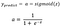Formula 3

It is worth saying that this a will be the final output that is the value in the y_train or y_test.

To define the sigmoid function in our code we add at the top:

About w and b being initialized as zeros let’s define a function to do it:

Cost function and Gradient descent

The cost function is a measure of a model that reflects how much the predicted output differs from the original output. The model aim to lower the cost function value and for that we use the following formula for all the rows.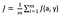Formula 4

Furthermore, we need to update the values of w and b in the formula 2. These values would be initialized as zeros but they will need more appropriate values and gradient descent will help us with that.

In Formula 4, the cost function is expressed as a function of a and y, but it can also be expressed as a function of w and b as well.

The differential w and b will be derived by taking the partial differentiation of cost function with respect to w and b.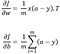Formula 5 and 6

# CODING THE MODEL

Once all the formulas are set, let’s put it all together in a function called propagate by adding the next lines in our code

This function calculates:

• A: Predicted output
• cost: Cost function
• dw and db: Gradients

By using this function now we are able to update w and b in Formula 2.

Optimize the parameters

To best fit the training data we will update the parameters which are the core of this model. The propagate function will be run through a number of iterations. In each iteration, w and b will be updated.

The term learning rate was introduced in this function. It’s not a calculated value. It can vary depending the different machine learning algorithms. Later in the conclusions I will show the impact it has.

Predict the output

By now we can optimize the parameters, reduce the cost function and update the values of gradients, so is the time to predict the output.

Model

By putting all the functions together the final model will look like this.

Using the model

To use the model and see how well it works let us pass our data that we prepared at the beginning by adding the next lines of code

Once the code it is executed it will appear on the console something like this

Percentages may vary depending on the number of iterations and learning rate you use.

Plotting data

Is worth noting the model function returns a dictionary that contains:

• Costs
• Final parameters
• Predicted Outputs
• Learning Rate
• Number of iterarions used

So, let us see how cost function changed with each updated ‘w’s and ‘b’s by adding the next lines of code.

After doing some tests changing values ​​I got the following results.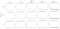Scatter Plot of Cost Functions with diferent learning rates and iterations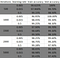Multiple values of test and train accuracy depending on learning rate and iterations

# CONCLUSIONS

• With each iteration, the cost function went down as it should, that means the parameters w and b kept refining towards perfection.
• It is worth noting that increasing the value of the learning rate will get us a better % at training accuracy but it will be lower on test accuracy. For this particular case I recommend using 0.015 that had both percentages more even.
• To change the model for recognizing another digit apart from 1 you can go to the line where it changes all numbers that are not 1 to zero, but instead of 1 use the digit of your preference.
• Reducing the number of rows for training the model will underfit it and if we increase the number it will overfit it. But you can try and check how it behaves.